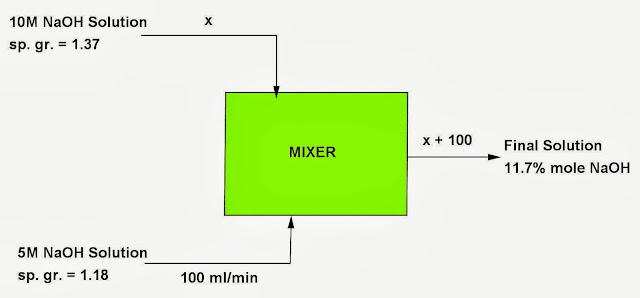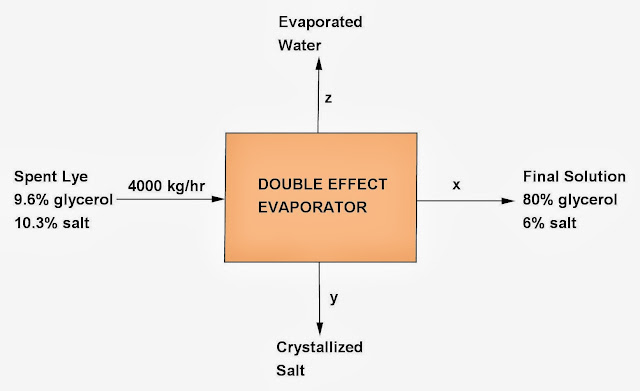## Monday, September 30, 2013

### Stoichiometry Problem - Material Balance, 20

Category: Chemical Engineering Math, Algebra

"Published in Newark, California, USA"

One hundred milliliters per minute of 5M NaOH solution (sp. gr. = 1.18) is mixed with 10M NaOH solution (sp. gr. = 1.37). It is desired to produce a solution containing 11.7 % mole NaOH. Calculate the required volumetric flow rate (ml/min) of 10M NaOH solution. Also, calculate the volumetric flow rate at the final solution. (Atomic Weights: Na = 23, O = 16, H = 1)

Solution:

The given word problem is about mixing of two NaOH solutions with different concentrations to produce a desired NaOH solution which involves the principles of Stoichiometry. The total amount of a substance in the reactants or incoming ingredients must be equal to the total amount of a substance in the final products. In short, the Law of Conservation of Mass must be followed all the time.  To illustrate the given problem, it is better to draw the flow diagram as followsPhoto by Math Principles in Everyday Life

Basis: 100 ml/min of 5M NaOH Solution

Let x = be the volumetric flow rate of 10M NaOH in ml/min

Molecular Weight Data:

NaOH = 40
H2O = 18

Consider 5M NaOH Solution:

Consider 10M NaOH Solution:

The total moles of NaOH from the two solutions is equal to

The total moles of H2O from the two solutions is equal to

Hence, the % mole of NaOH is equal to

Material Balance of NaOH:

Therefore, the volumetric flow rate of 10M NaOH Solution is 78.2 ml/min.

The volumetric flow rate of Final Solution is 100 ml/min + 78.2 ml/min = 178.2 ml/min

## Sunday, September 29, 2013

### Stoichiometry Problem - Material Balance, 19

Category: Chemical Engineering Math, Algebra

"Published in Newark, California, USA"

A spent lye sample obtained from a soap manufacturing unit is found to contain 9.6% glycerol and 10.3% salt (NaCl). It is concentrated at a rate of 4000 kg/hr in a double effect evaporator system until the final solution contains 80% glycerol and 6% salt. Assume that 5% glycerol is lost by entrainment. All percentages are by weight. Calculate the evaporation taking place in the system and the amount of salt that is crystallized in the salt box of the evaporator.

Solution:

The given word problem is about the evaporation of spent lye sample in a double effect evaporator which involves the principles of Stoichiometry. The total amount of a substance in the reactants or incoming ingredients must be equal to the total amount of a substance in the final products. In short, the Law of Conservation of Mass must be followed all the time.  To illustrate the given problem, it is better to draw the flow diagram as followsPhoto by Math Principles in Everyday Life

Basis: 4000 kg/hr of Spent Lye Sample

Let x = be the amount of Final Solution
y = be the amount of Crystallized Salt
z = be the amount of Evaporated Water

Overall Material Balance of Double Effect Evaporator:

Since the problem says that "5% glycerol is lost by entrainment", then the amount of glycerol at the Final Solution will be equal to

When glycerol becomes more concentrated, then it becomes more viscous. If it is more viscous, then it is hard to collect completely the concentrated glycerol from the container, mixer, or tank.

Material Balance of Glycerol:

Material Balance of Salt:

Substitute the values of x and y to the first equation, we have

Therefore,

Amount of Crystallized Salt = 384.5714 kg/hr
Amount of Evaporation = 3158.2857 kg/hr

## Saturday, September 28, 2013

### Stoichiometry Problem - Material Balance, 18

Category: Chemical Engineering Math, Algebra

"Published in Suisun City, California, USA"

The gaseous mixtures with the following composition expressed in % mole are to be blended as follows:Photo by Math Principles in Everyday Life

The three gas mixtures are to be blended in such a proportion that the final mixture should have 40% CH4, 35% C2H6, and 25% C3H8 (on mole basis). Calculate the proportion in which they should be mixed.

Solution:

The given word problem is about the mixing of gasses with different compositions which involves the principles of Stoichiometry. The total amount of a substance in the reactants or incoming ingredients must be equal to the total amount of a substance in the final products. In short, the Law of Conservation of Mass must be followed all the time. Since all gasses have the same type and compostion, then there's no chemical reactions involved in the mixture. To illustrate the given problem, it is better to label further the flow diagram as followsPhoto by Math Principles in Everyday Life

Basis: 100 kmole of Final Mixture

Let x = be the amount of Gas I
y = be the amount of Gas II
z = be the amount of Gas III

Overall Material Balance of Gas Mixer:

Material Balance of CH4:

Substitute the value of x to the first equation, we have

Material Balance of C2H6:

Substitute the value of x to the first equation, we have

Substitute the value of y to the third equation, we have

The value of y will be equal to

The value of x will be equal to

Therefore, the proportion of gas mixtures to be blended: